Next: The Crank-Nicholson scheme Up: The diffusion equation Previous: An example 1-d solution

## von Neumann stability analysis

Clearly, our simple finite difference algorithm for solving the 1-d diffusion equation is subject to a numerical instability under certain circumstances. Let us try to establish when this instability occurs. Consider the time evolution of a single Fourier mode of wave-number: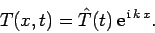(204)

Substitution of the above expression into our finite difference scheme (197) yields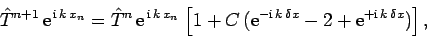(205)

or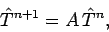(206)

where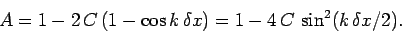(207)

Thus, the amplitude of the Fourier mode is amplified by a factorat each time-step. In order for the differencing scheme to be stable, the modulus of this amplification factor must be less than unity for all possible values of. Now, the largest possible value of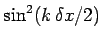is unity: hence, the wave-length corresponding to this value is that of the most unstable Fourier mode. In fact, the most unstable mode possesses a wave-length which is half the grid-spacing: i.e.,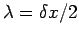. It follows from Eq. (207) that this mode is stable provided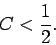(208)

Finally, from the definition of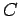, our stability condition can be written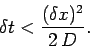(209)

Note that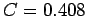for the stable calculation shown in Fig. 71, whereas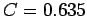for the unstable calculation shown in Fig. 72. Incidentally, the type of stability analysis outlined above is called von Neumann stability analysis. Note that the neglect of the spatial boundary conditions in the above calculation is justified because the unstable modes vary on very small length-scales which are typically of order the grid spacing.

According to Eq. (209), our finite difference scheme for solving the 1-d diffusion equation is only stable provided that the time-step remains below some critical value. Note that this critical value scales like the square of the grid-spacing. This is a very unfavorable scaling, since it implies that a doubling of the spatial resolution requires a simultaneous reduction in the time-step by a factor of four in order to maintain numerical stability. Certainly, the above constraint limits us to absurdly small time-steps in high resolution calculations. Is there any way of overcoming this constraint?Next: The Crank-Nicholson scheme Up: The diffusion equation Previous: An example 1-d solution
Richard Fitzpatrick 2006-03-29